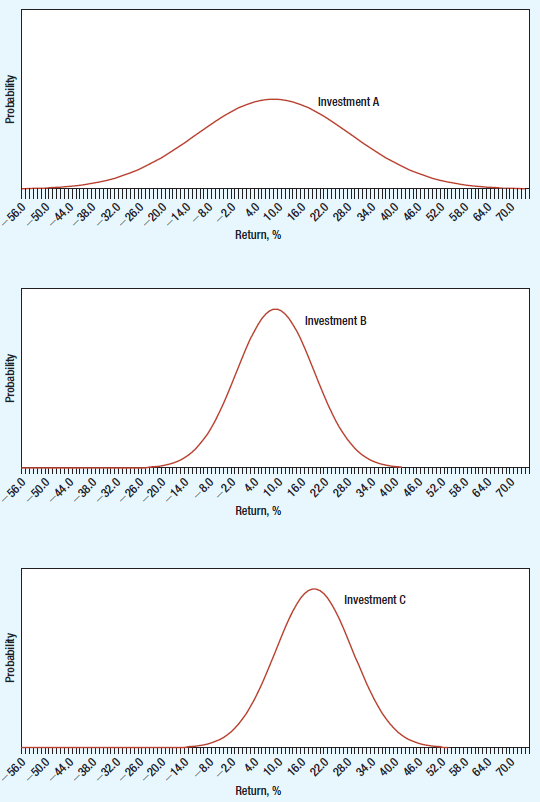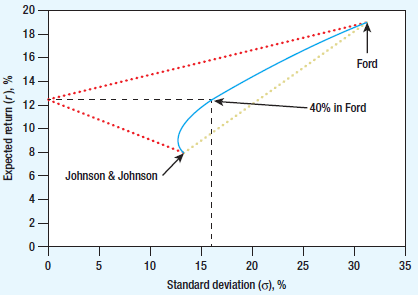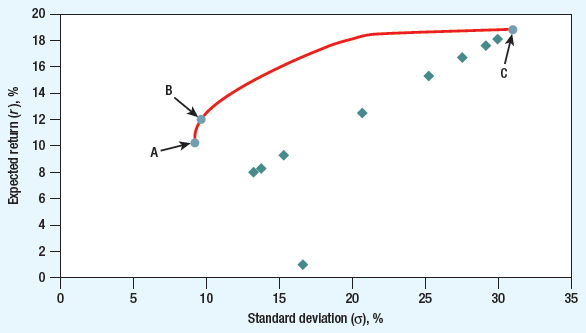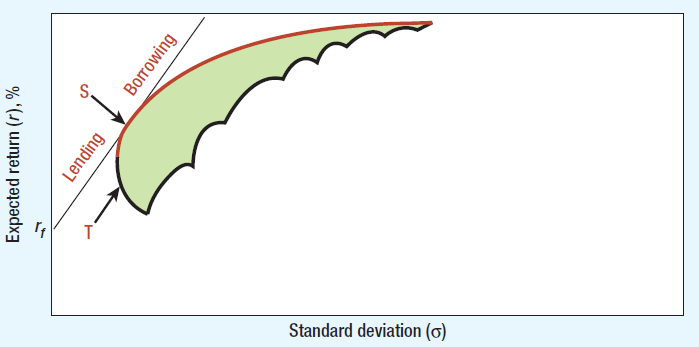# Sharpe Ratio Formula Calculator

Created by Tibor Pal, PhD candidate
Reviewed by Bogna Szyk
Based on research by
Brealey, R., Myers, S., Allen, F. Principles of Corporate Finance Mc Graw Hill Education (2016)
Last updated: Jul 10, 2022
The Sharpe ratio formula measures the excess return (or risk premium) per unit of deviation in an risky investment, thus helping you understand the return of an investment compared to its risk.

Sharpe ratio formula calculator helps you find out the attractiveness of a risky investment. In other words, the Sharpe ratio of risky investment is equal to the volatility of net worth. The concept of the Sharp ratio calculation is firmly connected to the Capital Asset Pricing Model (CAPM) that helps you find out what is the expected return of your asset or investment according to its inherent risk level.

## Conducting Sharpe ratio calculation

To understand the background of the Sharpe ratio formula, as the first step we need to get some insights in the parameters it uses.

The Sharpe ratio formula is the following:

Sharpe ratio = Risk Premium / σ

risk premium = Ra - Rf

where:

• Risk premium is the additional return that an investor requires to hold a risky asset rather than one that is risk-free. The CAPM calulator provides more insight for measuring risk premium together with some more explanation of risky and risk-free assets.
• Ra is the return of asset or investment.
• Rf is the risk-free return.
• σ or sigma stands for the standard deviation of a risky asset. A risky asset or investment means that it can have numerous possible outcomes. The usual measure of the spread of these possible outcomes is the standard deviation or variance. To see the statistical representation of the standard deviation, you may have a look at binomial distribution calculator.

To explain the variable sigma or standard deviation in a financial context, we aligned the following illustration that gives you more insight in a visual way. The three graphs display three different investments of possible returns.Source: Brealey, R., Myers, S., Allen, F. (2016). Principles of Corporate Finance. Mc Graw Hill Education

Investment A and B offer an expected return of 10%, but A has a much wider spread of possible outcomes. Its standard deviation is 15%; the standard deviation of B is 7.5%. Investors, generally, avert uncertainty and would prefer B to A. Comparing B to C, the standard deviation is the same; however, the expected return is 20% from C, and only 10% from B. Most investors prefer high expected return and would, therefore, prefer C to B.

The following graph shows how the expected return and standard deviation change if you decide to set up a simple portfolio by holding a different combination of two stocks. The curved blue line represents the expected return and risk that you could achieve by various combinations of the two stocks. For example, if you invest 40% of your money in Ford and the remainder in Johnson & Johnson, your expected return is 12.3%, which is 40% of the way between the expected returns on the two stocks. The standard deviation is 15.9%, which is less than 40% of the way between the standard deviations of the two stocks. It is because diversification reduces risk.Source: Brealey, R., Myers, S., Allen, F. (2016). Principles of Corporate Finance. Mc Graw Hill Education

However, in the real world, you are not limited to invest only in two stocks. Therefore the number of combinations are immense. In the below graph the spots represent different stocks with their expected return and standard deviation. There are many possible combinations of expected return and standard deviation from investing in a mix of these stocks. Like a reasonable investor, most probably you prefer high expected returns and dislike high standard deviations, so you would prefer portfolios along the red line. These are the efficient portfolios.Source: Brealey, R., Myers, S., Allen, F. (2016). Principles of Corporate Finance. Mc Graw Hill Education

Taking into account the possibility of lending and borrowing further extends the range of investments possibilities. On the following graph, the red line represents the scope of efficient portfolios.Source: Brealey, R., Myers, S., Allen, F. (2016). Principles of Corporate Finance. Mc Graw Hill Education

To find the most efficient one, you need to draw the steepest straight line from rf to the curved red line of efficient portfolios. That line will be tangent to the red curve. The efficient portfolio at the tangency point is the best choice as it gives you the highest expected return with the lowest standard deviation. However, also, it offers the highest ratio of risk premium to standard deviation. So probably you already know that the Sharpe ratio formula represent this ratio which is the tangency point on the red curve.

Tibor Pal, PhD candidate
Return of the asset or investment
%
Risk free return
%
%
Standard deviation
%
Sharpe ratio
People also viewed…

### Christmas tree

Welcome to the Christmas tree calculator, where you will find how to decorate your Christmas tree in the best way. Take a look at the perfect Christmas tree formula prepared by math professors and improved by physicists. Plan in advance how many lights and decorations you'll need!

### Liquidity coverage ratio (LCR)

Our LCR calculator helps you to calculate the liquidity position of a bank in a stress scenario.

### Mortgage Payoff Calculator

Use the Mortgage Payoff Calculator to follow the payoff schedule of either an existing or a new mortgage loan with the option of adding an extra monthly payment or single repayment.

### Social Media Time Alternatives

Check what you could have accomplished if you get out of your social media bubble.